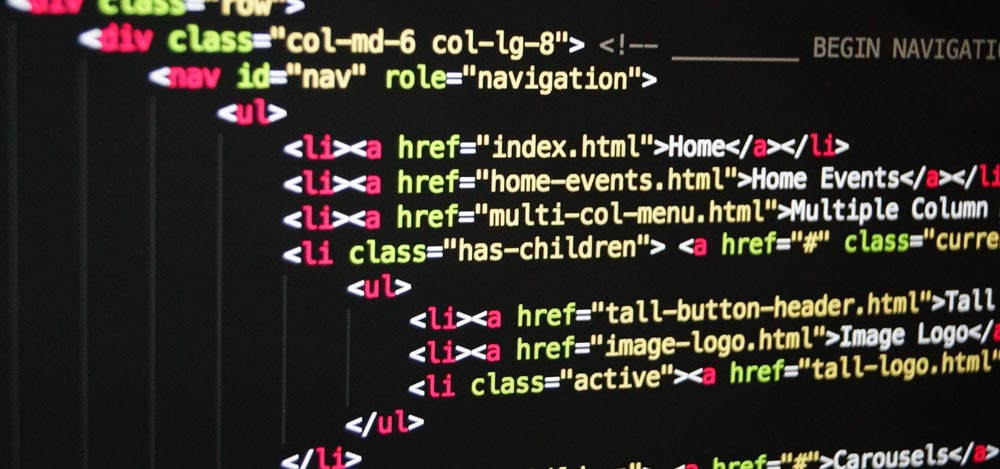# Combining arrays in PHPIf you need to combine two arrays in PHP, you first thought might be to use the `+` operator. However, this is unlikely to do what you want it to to. If we look at the following example, you might expect it to output an array with all three elements.

```<?php

\$listA = ['banana'];
\$listB = ['apple', 'pear'];
\$listC = \$listA + \$listB;

var_dump(\$listC);```

However, what you will actually get back is an array containing two elements: banana and pear. This is because when you use the + operator in PHP it combines it using array keys. Even though these are non-indexed arrays, PHP looks at them like this:

```[0 => 'banana']
[0 => 'apple', 1 => 'pear']```

Therefore when you add them together, it combines the keys, taking the earliest. In this case, to get the result you want, you want to use the `array_merge` function. For other scenarios, PHP has a range of different functions to combine arrays in different ways.

Timeline